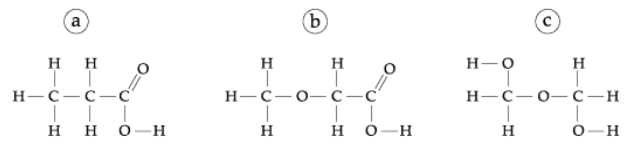# Problem: Which of the following could be the structure of C3H6O3?Organic compounds Organic formulas follow the convention of placing C first in the formula, followed by H, followed by the remaining symbols in alphabetical order.

###### FREE Expert Solution
79% (224 ratings)View Complete Written Solution
###### Problem Details

Which of the following could be the structure of C3H6O3?Organic compounds

Organic formulas follow the convention of placing C first in the formula, followed by H, followed by the remaining symbols in alphabetical order.